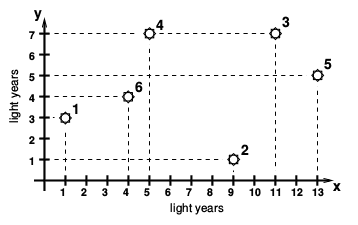### G - Galaxy collision

##### Languages: C, C++, Java, Pascal, ... (details)

The Andromeda galaxy is expected to collide with our Milky Way in about $3.8$ billion years. The collision will probably be a merging of the two galaxies, with no two stars actually colliding. That is because the distance between stars in both galaxies is so huge. Professor Andrew is building a computational model to predict the possible outcomes of the collision and needs your help! A set of points in the two dimensional plane is given, representing stars in a certain region of the already merged galaxies. He does not know which stars came originally from which galaxy; but he knows that, for this region, if two stars came from the same galaxy, then the distance between them is greater than $5$ light years. Since every star in this region comes either from Andromeda or from the Milky Way, the professor also knows that the given set of points can be separated into two disjoint subsets, one comprising stars from Andromeda and the other one stars from the Milky Way, both subsets with the property that the minimum distance between two points in the subset is greater than $5$ light years. He calls this a good separation, but the bad news is that there may be many different good separations. However, among all possible good separations there is a minimum number of stars a subset must contain, and this is the number your program has to compute.For example, the picture illustrates a given set of six points. Professor Andrew cannot tell which stars came from Andromeda, but note that there are four possible good separations: $\{\{1, 2, 4, 5\}, \{3, 6\}\}$; $\{\{1, 2, 3, 4\}, \{5, 6\}\}$; $\{\{1, 4, 5\}, \{2, 3, 6\}\}$; $\{\{1, 3, 4\}, \{2, 5, 6\}\}$. Therefore, at least two stars must have come from Andromeda, since this is the minimum number of points a subset may have in a good separation.

#### Input

The first line contains an integer $N$ ($1 \leq N \leq 5 \times 10^4$) representing the number of points in the set. Each of the next $N$ lines describes a different point with two integers $X$ and $Y$ ($1 \leq X, Y \leq 5 \times 10^5$), indicating its coordinates, in light years. There are no coincident points, and the set admits at least one good separation.

#### Output

Output a line with an integer representing the minimum number of points a subset may have in a good separation.

#### Sample test(s)

Input
6 1 3 9 1 11 7 5 7 13 5 4 4
Output
2
Input
2 10 10 50 30
Output
0